Next: Pauli Two-Component Formalism Up: Spin Angular Momentum Previous: Magnetic Moments

# Spin Precession

The Hamiltonian for an electron at rest in a-directed magnetic field,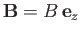, is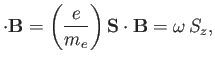(5.48)

where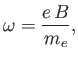(5.49)

and use has been made of Equation (5.47). According to Equation (3.28), the time evolution operator for this system is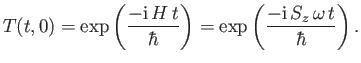(5.50)

It can be seen, by comparison with Equation (5.24), that the time evolution operator is precisely the same as the rotation (about the-axis) operator for spin, with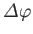set equal to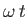. It is immediately clear that the Hamiltonian (5.48) causes the electron spin to precess about the-axis with angular frequency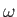. In fact, Equations (5.35)-(5.37) imply that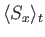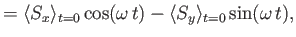(5.51)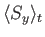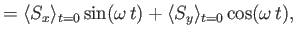(5.52)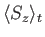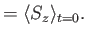(5.53)

Note that the expectation value of the electron spin precesses in a right-handed fashion. In other words, if the thumb of the right hand is directed along the magnetic field then the fingers of the right-hand indicate the direction of the precession. A particle with a positive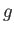-factor would precess in a left-handed fashion. The time evolution of the state ket is given by analogy with Equation (5.40):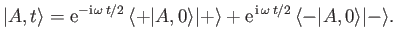(5.54)

Note that it takes a time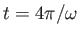for the state ket to return to its original state. By contrast, it only takes a time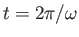for the spin vector to point in its original direction.

We shall now describe an experiment to detect the minus sign in Equation (5.41). An almost mono-energetic beam of neutrons is split in two, sent along two different paths, I and II, and then recombined. Path I passes through a magnetic-field-free region. However, path II enters a small region where a static magnetic field is present. As a result, a neutron state ket going along path II acquires a phase-shift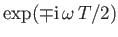(the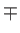signs correspond to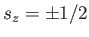states). Here,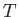is the time spent in the magnetic field, and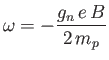(5.55)

is the spin precession frequency. Moreover,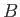is the magnetic field-strength,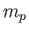the proton mass, and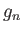the neutron-factor. This factor is found experimentally to take the value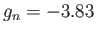. (The magnetic moment of a neutron is entirely a quantum field effect. Incidentally, this particular experiment must be performed with electrically neutral particles because, otherwise, the particles that pass through the magnetic field would be strongly perturbed by the Lorentz force.) When neutrons from path I and path II meet they undergo interference. We expect the observed neutron intensity in the interference region to exhibit a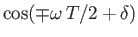variation, where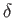is the phase difference between paths I and II in the absence of a magnetic field. In experiments, the time of flightthrough the magnetic field region is kept constant, while the field-strengthis varied. It follows that the change in magnetic field required to produce successive maxima is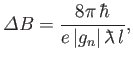(5.56)

whereis the path-length through the magnetic field region, and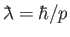is the reduced (by a factor) de Broglie wavelength of the neutrons. The previous formula has been verified experimentally to within a fraction of a percent [89,116]. Note that the formula in question depends crucially on the fact that it takes a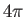rotation to return a state ket to its original state. If it only took arotation then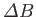would take half of the value given in Equation (5.56), which does not agree with the experimental data.Next: Pauli Two-Component Formalism Up: Spin Angular Momentum Previous: Magnetic Moments
Richard Fitzpatrick 2016-01-22